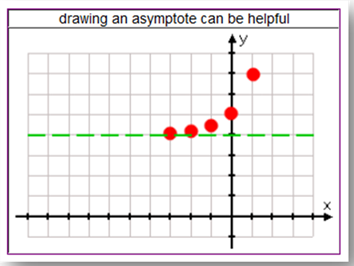Asymptotes

An asymptote is a line that a curve approaches but never crosses. The asymptote for all of the curves presented in this book so far is the line y = 0, since the curves approach the x-axis, but do not touch or cross the line. This is also called the horizontal asymptote. Sometimes making a dotted line to represent the asymptote will help to draw the curve more accurately.

The graph below represents the function, y = 2x + 4. Notice that the points on the graph are approaching, but never touching, the line y = 4. Therefore, y = 4 is the asymptote line for this function.Notice in the graph above that the y-intercept is not equal to the a value of the equation. When a value is added to an exponential equation, the curve is shifted. This situation will be discussed in the "Transformations" book later in this unit.

Listen